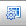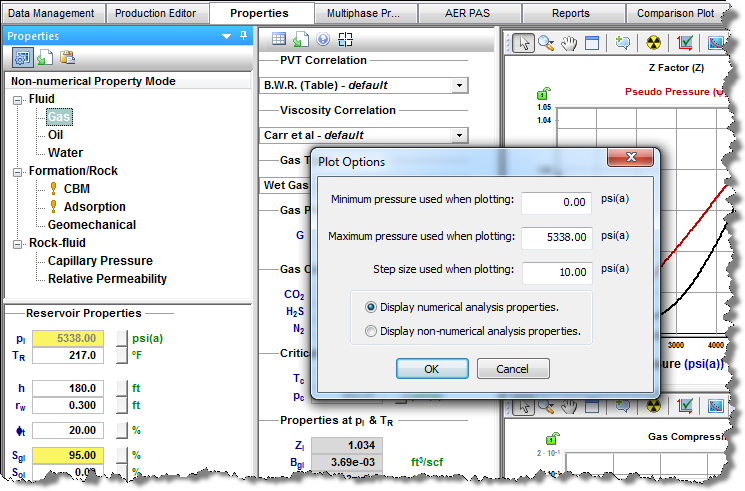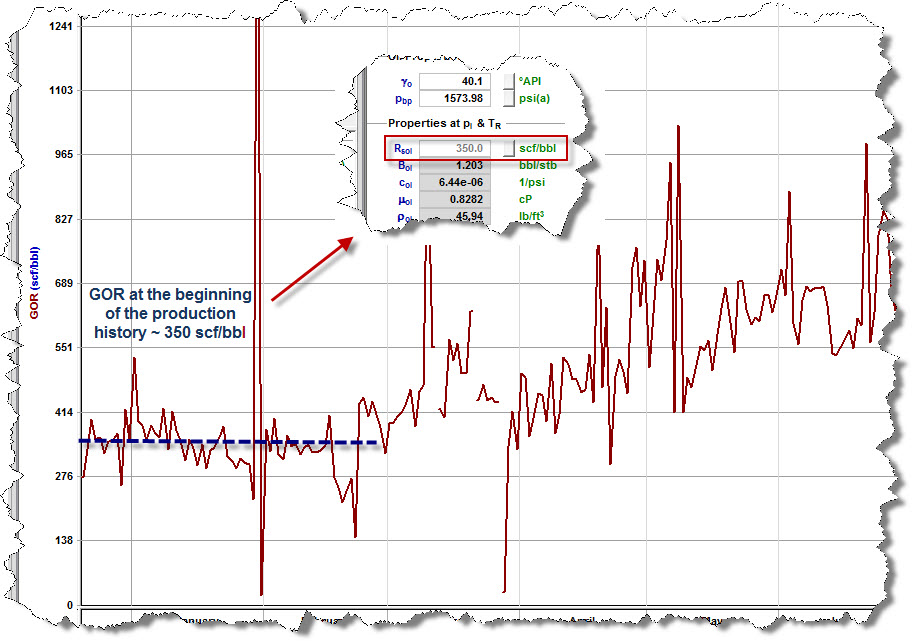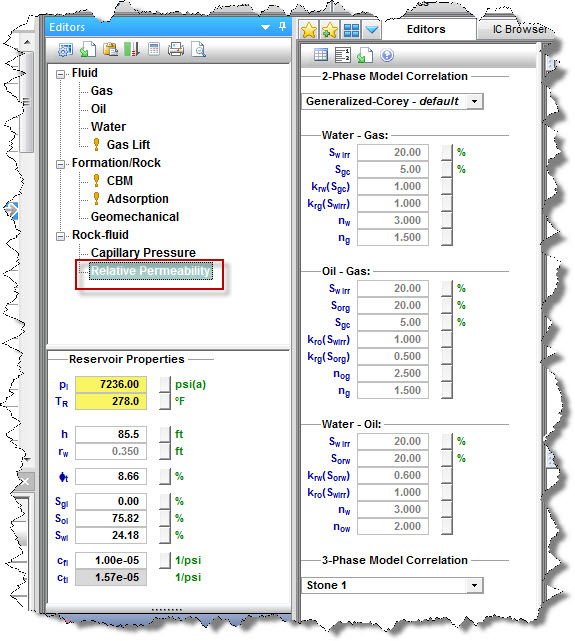# Advanced Numerical Models

Subtopics:

Related topics:

With WellTest, you can access robust multiphase reservoir models with rectilinear gridding. The model is not designed to perform as a complete full-field reservoir simulator, but rather as a history matching and forecasting tool for single well performance. The model is deployed in practical configurations as follows:

• Advanced Vertical Numerical Model
• Advanced Fracture Numerical Model
• Advanced Horizontal Numerical Model
• Advanced Horizontal Multifrac Numerical Model
• Advanced Extended Hz Multifrac Numerical Model

Each of these configurations can be launched for single-phase (gas or water), two-phase (gas-oil or gas-water), or three-phase (gas-oil-water) systems. Additionally, you can model gas condensate and volatile oil systems with or without accounting for water.

## Use of Numerical Models

Most well performance analysis and modeling can be accomplished using analytical models, including cases with complex geometry, such as multiple fractures, heterogeneities, and non-symmetrical reservoir boundaries. However, there are cases where gridded numerical models are useful and/or required.

Numerical models serve three important purposes:

1. Validation of Analytical models — Numerical models are used to ensure that complex analytical models exhibit expected behaviours. It should be noted that analytical and numerical solutions never yield exactly the same answer. However, for a successful validation case, the difference ought to be less than 10% when comparing cumulative production over a fixed period, or when comparing simulated pressure responses to a defined rate history. (In some very complex cases, such as multi-frac’d shale gas completions with pressure-dependent permeability and/or adsorbed gas, we have noted greater than a 10% difference between the analytical and numerical solutions. Thus, the numerical models provide a valuable backup, in the event that the analytical models have been stretched beyond their capabilities.) In general, it is a good idea to approach well performance analysis from several independent perspectives. Agreement between analytical and numerical models usually leads to the most robust interpretation possible.

2. History matching and forecasting multiphase systems — Numerical models are ideal for modeling multiphase situations such as solution gas or gas condensate. In order to adequately account for the complexities of multiphase flow, reservoir heterogeneity and the mechanisms responsible for flow, the model must be able to account for capillary and gravity effects, and handle spacial and temporal changes in fluid saturation, rock and fluid properties, and relative permeability. Analytical solutions are very difficult to obtain because these phenomena are highly non-linear. Numerical solutions, on the other hand, require fewer simplifying assumptions for reservoir properties.  The nonlinear partial-differential equations (PDEs) are solved by dividing the reservoir into smaller blocks, discretizing the PDEs into algebraic equations, and performing rigorous material balance calculations on each block. The solutions describe the pressure and phase saturation at discrete points in the reservoir at discrete times.

3. Visualization of dynamic reservoir properties — Analytical models are limited in that they only provide the pressure / rate solution at the sandface. In order to visualize how pressures and saturations are changing throughout the reservoir, gridded numerical models are required. With numerical models, you can view, dynamically through time, pressure or saturation as a 2-dimensional color gradation map, or as a cross-section.

## Prerequisites

Before you start modeling, ensure that all required information is added to the WellTest project. Well production data (e.g., rates and sandface pressures) should be populated in the Production Editor Tab.

We recommend that you create and match an analytical model prior to numerical modeling. This gives you an idea as to which reservoir and completion parameters to use, and makes it easier to history match.

To create a numerical model that accurately captures the reservoir behaviour, it is essential that the fluid properties are populated correctly. See the section below for details.

## Properties for Numerical Modeling

Fluid and rock properties for the well are stored in the Properties Tab.

### Gas Properties for Numerical Modeling

To set gas properties for numerical modeling, set the Gas Type to Dry Gas, Wet Gas, or Liquid-Rich Gas.

To be able to run a Gas Condensate or Volatile Oil numerical model for the well, you must select Liquid-Rich Gas as a gas type. For a additional information, see Differences between Black Oil, Gas Condensate, and Volatile Oil Numerical Models.

Important: If the Gas Type is Liquid-Rich Gas, properties used for numerical modeling are slightly different from the properties used for other types of modeling and analysis. To be able to display and edit properties used for numerical modeling, click the Plot Options icon () on the toolbar and select the Display numerical analysis properties option in the Plot Options dialog box.If you select Liquid-Rich Gas as the Gas Type, you have to set the Vaporized Oil Ratio (Rv). If you have lab data available, you can set Rv as a table. Alternatively, you can use the correlation suggested by Ovalle et al.

### Oil Properties for Numerical Modeling

If you know the value for the oil bubble point, enter it to the pbp field.

If you do not have this information, and you believe that the well is operating above the bubble point for at least the beginning of the production history, we recommend the following workflow:

1. Estimate the gas-oil ratio (GOR) at the beginning of the production history (when the GOR is fairly flat).

2. Enter your estimate as the Solution GOR (Rsol). (Pbp is calculated based on Rsol.)By following this workflow, you ensure that the modeled GOR matches the production GOR (at least at the beginning of the production).

### Relative Permeability

For accurate numerical modeling of the multiphase system, relative permeabilities should be set.

By default, relative permeabilities use the Generalized-Corey correlation with preset default parameters. If you have relative permeability data (from lab studies, or based on an analog well), we recommend that you adjust correlation parameters, or set permeabilities as a custom table.